# Partial fraction decomposition online calculator

Rational fraction R(x) is called the fraction of the form: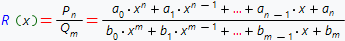If n<m, fraction is called proper. Common fractions is the rational fractions of the form:, where

n, m - natural numbers, coefficients c, p, q, A, B, C are real numbers and the roots of the polinomial x2+px+q - is complex conjugate (i.e. ¼p2q<0).

If the denominator is decomposed Qm(x) - in the multiplication of the linear and/or quadratic multipliers:

Qm(x) =(xc1) n1 ... (xcr) nr ... (x2+p1x+q1 ) m1 ... (x2+psx+qs ) ms , where

c1, c2, ..., cr - real roots of the polinomial Qm(x) of order n1, n2, ..., nr respectively, and x2+pkx+qk =(xak) (xãk), where ak и ãk complex conjugate roots of order mk, then initial fraction can be respesented such as:Each linear multiplier of the form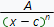, contained in Qm(x) corresponds the decomposition of the form: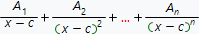Each quadratic multiplier of the form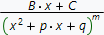, contained in Qm(x) corresponds to the decomposition of the form: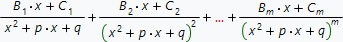Our online calculator finds partial fraction decomposition of any (proper, improper) rational fraction. If initial fraction is the improper one, (i.e. order of polynomial in the numerator greaters of equals to the order of polynomial in the denominator) calculator divides numerator to the denominator and extracts the proper fraction. Partial fraction decomposition is usually used to find the integrals of the rational expressions.

The example of the step by step solution is here.

## Choose expression input type:

Expression input type:

## Choose options to find partial fraction decomposition:

Function variableR(x)=